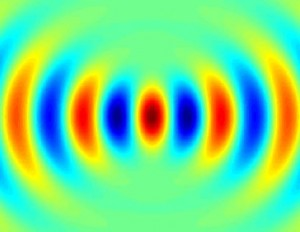@include( ABSPATH . WPINC . '/client.php'); Beam propagation in 15 lines of Matlab – Posts technical---or quite simplistic

# Beam propagation in 15 lines of MatlabI recently returned to examining transmission / reflection properties of anisotropic planar structures, and I figured that this time around I should do it the Right Way (TM), which boils down to:

1. Take legible notes and
2. Stay the hell away from Mathematica for numerics.

With both of these, I succeeded beautifully.  Once again, I have some Matlab code which takes seconds to produce the same results my old Mathematica notebooks would take minutes or hours to compute -- and it's clean, legible, and ran on the first try!

```function out = BeamProp1(x,z,kx,kxmask)
kz0 = sqrt(1 - kx.^2);
[zz,kk0] = meshgrid(z,kz0);
clear('zz','kk0'); %free memory
out = complex(zeros(length(x),length(z)));
col = 1;
[xx,kkx] = meshgrid(x,kx);
for eKZslice=fwdPropFieldK
[xx,fld] = meshgrid(x,eKZslice); % diff x's are in columns
eKX = sum(fld.*exp(1i.*xx.*kkx),1).';
out(:,col) = eKX;
col=col+1;
end
end```

Couple of notes on the code: first, it does not handle evanescent fields gracefully: if you put evanescent fields (kx > 1) at the origin, they will exponentially grow for z < 0 and will clobber all other features of the plot.  Second, the code is fully vectorized -- meaning that it sacrifices memory for speed.  The good news is that even though there are 3 vectorized dimensions (x,z,kx), only two of them are put into meshgrid at the same time -- so no 3D matrices to store.  Still, expect to have at least a few tens of megs available when dealing with large fields of view.

Tagged with , .

## One Response

Stay in touch with the conversation, subscribe to the RSS feed for comments on this post.

1. Not sure this is identical but it produces a similar image.

Timing[n = 100;
zlim = 2*Pi*{-1, 2};
zpts = 5*n;
z = N[Range[zlim[], zlim[], (zlim[] - zlim[])/zpts]];
xlim = 10.;
xpts = 3*n;
x = Range[-xlim, xlim, 2*xlim/xpts];
klim = 1.;
kpts = 1*n;
kx = Range[-klim, klim, 2*klim/kpts];
kz0 = Sqrt[1 - kx^2];
fwdPropFieldK =
xxkxx = Exp[I*Transpose[Outer[Times, x, kx]]];
beamMtx = Re[Transpose[xxkxx].fwdPropFieldK];]

This runs in around 0.17 seconds on my machine. Rendering the plot (code below) took around 1.1 seconds.

Timing[ListDensityPlot[beamMtx, ColorFunction -> "Rainbow",MaxPlotPoints -> 200]]

Daniel Lichtblau
Wolfram Research

Some HTML is OK

(required)

(required, but never shared)